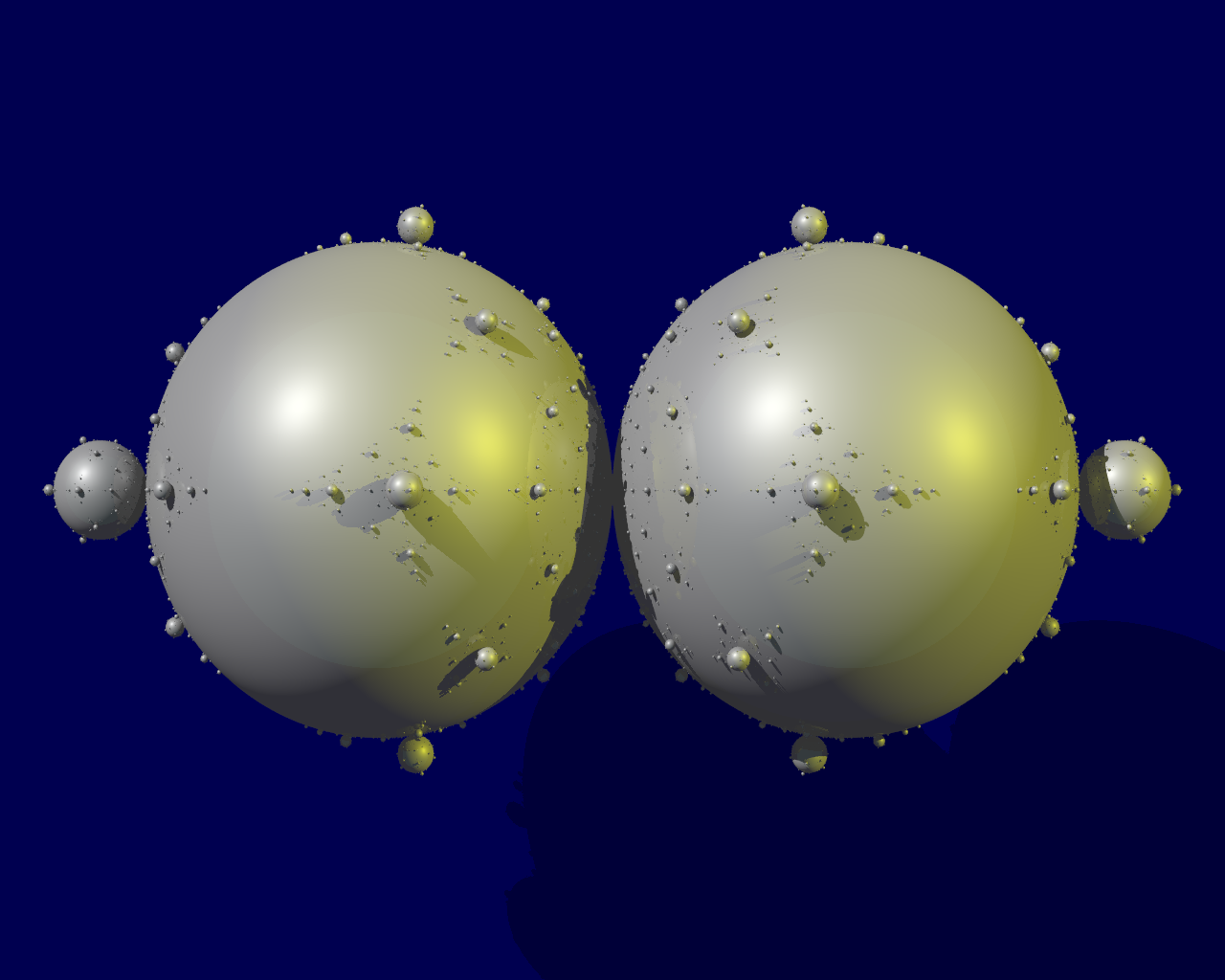doc. RNDr. Václav Kučera, Ph.D. Sokolovská 83 186 75 Praha 8 - Karlín Czech Republic Tel. :   [+420] 9 5155 3362 e-mail: kucera@karlin.mff.cuni.czVáclav Kučera Charles University Prague, Czech Republic

# Research## Main scientific results

• Robust numerical scheme for low Mach number (10-6) as well as transonic and supersonic compressible flows .
• Robust numerical scheme for fluid structure interactions using the ALE method .
• Optimal error estimates for DG applied to a nonlinear convection and nonlinear diffusion problem .
• Analysis of a space-time DG method for nonlinear convection-diffusion .
• Simulation of voice formation in human vocal chords .
• Error estimates uniform w.r.t. diffusion for DG for nonlinear convection-diffusion and purely convective problems .
• "Nice" triangles cannot be subdivided into "ugly" triangles only. .
• The circumradius condition (estimate) is a simple scaling of the standard maximum angle condition .
• Development of a numerical method for cell segmentation based on the level set method .
• Efficient numerical scheme for a nonlocal hydrodynamic model of flocking dynamics .
• Generalization of known sufficient conditions for FEM convergence [preprint].
• Formulation of a necessary condition for FEM convergence, construction of various counterexamples [preprint].
• Error estimates uniform w.r.t. time for DG for advection-diffusion problems .
• Proof of consistency with the low Mach incompressible limit for a general class of linearized schemes for the compressible Euler equations .
• "Nice" tetrahedra cannot be subdivided into "ugly" tetrahedra only .
• Generalization of Godunov's numerical flux to network junctions .

## Ongoing work

Currently I am digressing from my usual field of numerical analysis into the world of 3D fractals and chaotic dynamical systems. This was always a hobby of mine and now thanks to the Neuron fund, I can finally start doing serious reserch. As a preview, here are some POV-Ray pictures of 2D Kleinian limit sets, their trivial extensions into 3D and nontrivial 3D extensions.